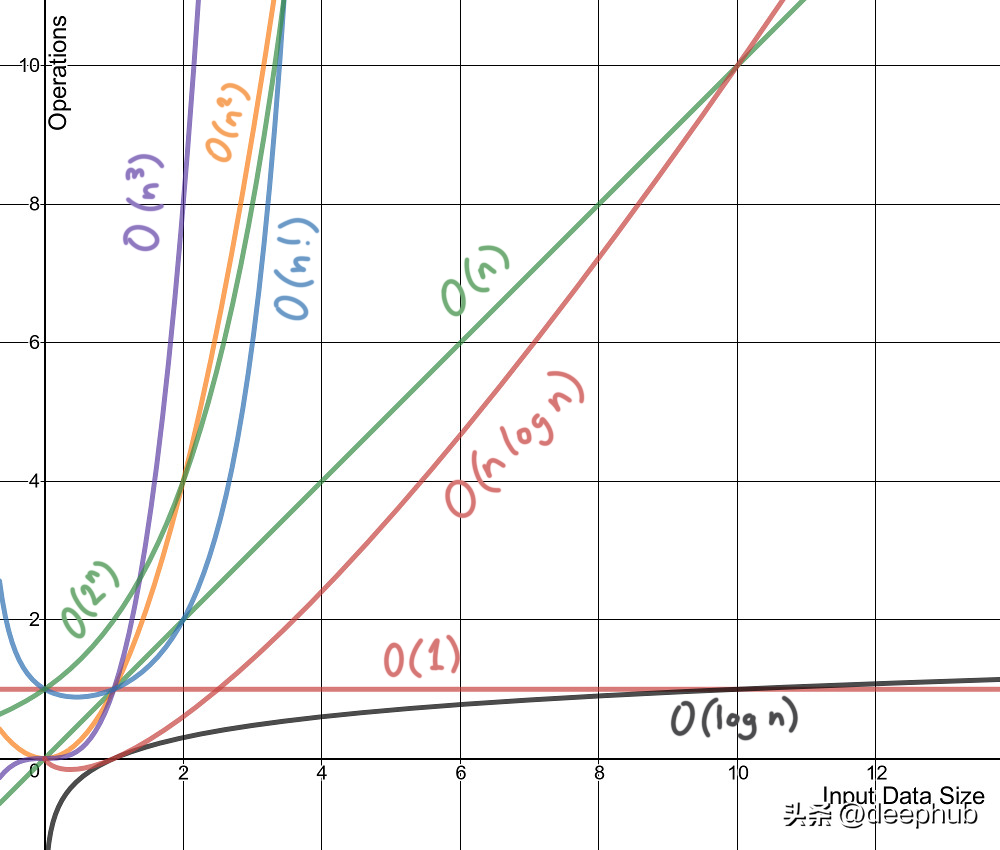## 八个常见的机器学习算法的计算复杂度总结

#### 1、时间复杂度#### 1、线性回归

• n= 训练样本数，f = 特征数
• 训练时间复杂度：O(f²n+f³)
• 预测时间复杂度：O(f)
• 运行时空间复杂度：O(f)

#### 2、逻辑回归：

• n= 训练样本数，f = 特征数
• 训练时间复杂度：O(f*n)
• 预测时间复杂度：O(f)
• 运行时空间复杂度：O(f)

#### 3、支持向量机：

• n= 训练样本数，f = 特征数，s= 支持向量的数量
• 训练时间复杂度：O(n²) 到 O(n³)，训练时间复杂度因内核不同而不同。
• 预测时间复杂度：O(f) 到 O(s*f):线性核是 O(f)，RBF 和多项式是 O(s*f)
• 运行时空间复杂度：O(s)

#### 4、朴素贝叶斯：

• n= 训练样本数，f = 特征数，c = 分类的类别数
• 训练时间复杂度：O(n*f*c)
• 预测时间复杂度：O(c*f)
• 运行时空间复杂度：O(c*f)

#### 5、决策树：

• n= 训练样本数，f = 特征数，d = 树的深度，p = 节点数
• 训练时间复杂度：O(n*log(n)*f)
• 预测时间复杂度：O(d)
• 运行时空间复杂度：O(p)

#### 6、随机森林：

• n= 训练样本数，f = 特征数，k = 树的数量，p=树中的节点数，d = 树的深度
• 训练时间复杂度：O(n*log(n)*f*k)
• 预测时间复杂度：O(d*k)
• 运行时空间复杂度：O(p*k)

#### 7、K近邻：

n= 训练样本数，f = 特征数，k= 近邻数

Brute：

• 训练时间复杂度：O(1)
• 预测时间复杂度：O(n*f+k*f)
• 运行时空间复杂度：O(n*f)

kd-tree：

• 训练时间复杂度：O(f*n*log(n))
• 预测时间复杂度：O(k*log(n))
• 运行时空间复杂度：O(n*f)

#### 8、K-means 聚类：

• n= 训练样本数，f = 特征数，k= 簇数，i = 迭代次数
• 训练时间复杂度：O(n*f*k*i)
• 运行时空间复杂度：O(n*f+k*f)
• 周排行
• 月排行
• 评论排行